俄罗斯方块

一.先定义俄罗斯方块中最小单位：方块    Cell类

I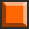O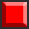S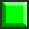Z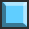T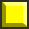L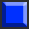J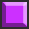public class Cell {
private int row;		//行
private int col;		//列
private BufferedImage image;		//图片
public Cell(int row, int col, BufferedImage image) {
super();
this.row = row;
this.col = col;
this.image = image;
}

public Cell() {
}

public int getRow() {
return row;
}
public void setRow(int row) {
this.row = row;
}
public int getCol() {
return col;
}
public void setCol(int col) {
this.col = col;
}
public BufferedImage getImage() {
return image;
}
public void setImage(BufferedImage image) {
this.image = image;
}
}

public void left() {
col--;
}
public void right() {
col++;
}
public void drop() {
row++;
}

二.四格方块共有的行为模式    Tetromino类

1）我们将四个小方块（cell）作为一个整体放入数组当中进行统一操作：

protected Cell[] cells = new Cell;

2）对作为整体的四格方块提供相应的行为方法，即四格方块左移/右移/软下落，其中的每个小方块都左移/右移/软下落，使用for循环对每个小方块都实现left()/right()/drop()方法，并提供toString方法：

	public void moveLeft() {				//四个方块向左移动
for(int i = 0;i<cells.length;i++) {
cells[i].left();
}
}

public void moveRight() {				//四个方块向右移动
for(Cell c:cells) {		//增强for循环
c.right();
}
}

public void softDrop() {				//四个方块向下移动
for(int i = 0;i<cells.length;i++) {
cells[i].drop();
}
}

@Override
public String toString() {
return "[" + Arrays.toString(cells) + "]";
}

3）定义一个静态方法randomOne()，实现四格方块的随机生成能力，期间用到Math.random()方法生成随机数，根据生成的不同随机数利用switch_case语句对不同的方块生成进行选择：

public static Tetromino randomOne() {
Tetromino t = null;
int num = (int)(Math.random()*7);
switch(num){
case 0:
t = new T();
break;
case 1:
t = new I();
break;
case 2:
t = new O();
break;
case 3:
t = new J();
break;
case 4:
t = new L();
break;
case 5:
t = new S();
break;
case 6:
t = new Z();
break;
}
return t;
}